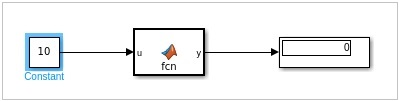In this chapter we will understand how to make use of MATLAB function in Simulink.

Open Simulink and click on blank model.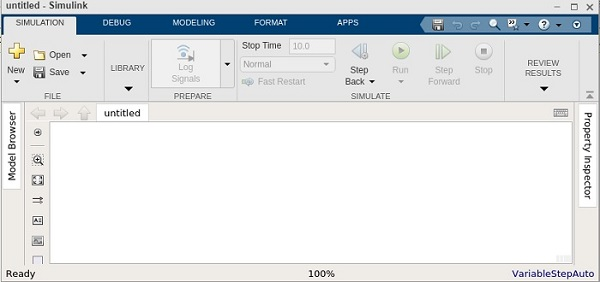We need a constant block, a MATLAB function block and a display block for the output.

Here is the model created −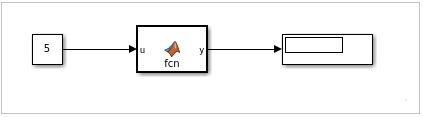The MATLAB function is available inside the user defined functions library.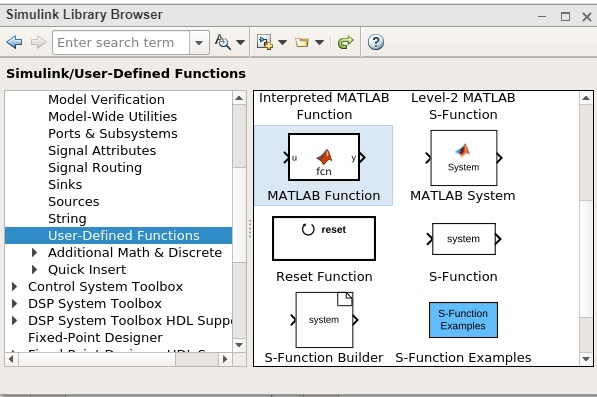Now, click on MATLAB function and drag it inside your model canvas. Double click on the MATLAB function block and write a function of your choice.

We will try to display 1 if the number given is odd and 0 if it is even. You can also save the function to be used later with another one.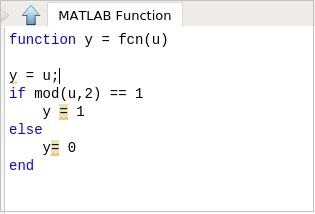Once the function is done click on the upward arrow close to the MATLAB function and it will take you back to the model you created earlier.

Now, let us test the model by using the constant value as 5.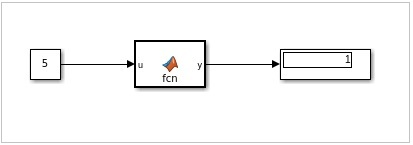We can see the display as 1, which indicates that the value 5 is an odd number.

Let us now change the constant value to 10 as shown below and run the simulation: The output is 0 indicating it is even number.